# Designing an Antenna for MAX66300

### Abstract

This application note examines designing an antenna for the MAX66300, which provides systems with a highly integrated NFC/RFID reader for contactless communication at 13.56MHz and a SHA-256 Secure Authenticator Coprocessor.

### Introduction

The purpose of this application note is to give more information on how to implement an antenna design needed for the MAX66300 NFC/RFID Reader and Authenticator IC.

### MAX66300 Analog Front-End Description

The MAX66300 analog front-end (AFE) integrates a transmission and reception chain compliant with all the 13.56MHz air interface standards.

In effect, the MAX66300 internal Configuration Word register (Option bits) permits setting the following parameters:

• Modulation index: 100% or 7% to 30%
• Data rate and data encoding, frames

• Subcarriers: 424kHz to 484kHz (ISO 15693). The user can adapt the reader chip internal filters to get the maximum performance.
• Data rate, data encoding, frames.

The different configurations, offered by the reader chip, that the MAX66300 supports:

• ISO 15693 standard

### MAX66300 Analog Front-End (Internal Structure)

The reader transmitter of the AFE, as shown in Figure 1, is composed by:

• Antenna drivers (push-pull structure)
• Modulator
• Oscillator driven by the external crystal or through an OSCIN input (option bit 26)

Using option bits 6 and 7, the AFE can support three different topographies to connect to an antenna such as:

• Direct antenna connection
• Double parallel output driver
• RFIN demodulation Inputs### Direct Antenna Connection

In the configuration of Figure 2, the antenna is directly connected to the reader outputs through a resonant capacitor.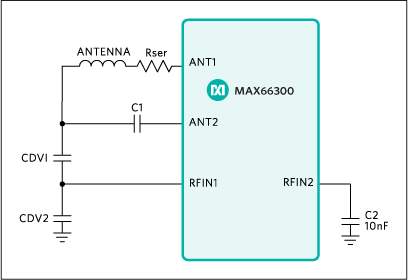For the antenna tuning, it is recommended to follow the example given below:

1. Measure the antenna parameters with a RLC meter at 13.56MHz.

• Antenna inductivity: 1.2µH
• Quality factor: 20

The ohmic antenna resistance can be found by applying the following formula: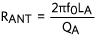RANT = 5.11Ω

2. Calculate the resonant capacitor value (CRES) as follows:

• f0 = 13.56MHz
• L = 1.2µH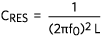CRES = 115pF

3. Calculate the series resistor in-line with the antenna as follows:

• RANT = 7Ω
• VDD = 5V
• VSS = 0V
• IANT (peak) = 141mARSER = 20Ω

This resistor is used to limit the current of the reader output stage drivers.

4. Calculate the voltage across the resonant capacitor to determine the capacitor divider as follows:
5.VANT = 20V

To suite the maximum specifications at RFIN inputs, the antenna voltage would have to be divided by nearly a factor of 4.

6. Lastly, calculate the capacitor values for Figure 2 using Cres formula given here:

• Cres = 115pF (calculated previously in step 2)
• DC capacitor divider would equal 4C1 = 100pF
CDV1 = 15pF
CDV2 = 68pF

### Double Parallel Output Driver

In case of a remote antenna configuration, as shown in Figure 3, a matching impedance circuitry is used to adapt the coaxial cable load to the reader output impedance. A Smith chart, as shown in Figure 4, is used to determine the component values of the matching network.As an example:

• Needed antenna impedance: 50Ω
• ANT1 and ANT2 output impedance: 3.5Ω (driving in phase)
 Reference Value C1 680pF, 0805, NPO technology C2 680pF, 0805, NPO technology C3 820pF, 0805, NPO technology C4 1nF, 0805, NPO technology C5 680pF, 0805, NPO technology C6 560pF, 0805, NPO technology C7 820pF, 0805, NPO technology C8 33pF, 0805, NPO technology L1 180nH, low serial resistance L2 270nH, low serial resistance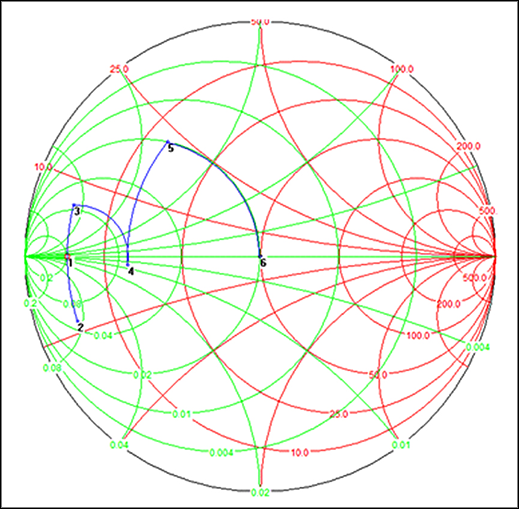To adapt from the 3.5Ω output driver resistance to the 50Ω antenna impedance load, six steps are used as shown in the following example:

• Step 1 uses the corresponding driver output impedance of 5Ω from the MAX33600.
• Step 2 obtains the impedance by adding the two serial capacitors of 680nF.

•• Step 3 adjusts the impedance outcome due to the effects of the serial inductor L1 to Z = 3.5 + 6.9j.

•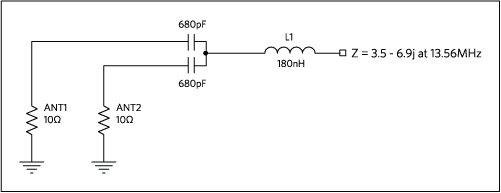• Step 4: The shunt capacitor network formed by C3, C4 and C5 has a global capacitance of 1.224nF which yields an impedance of 14.6 – 1.1jΩ for this example.

•• Step 5: The second serial inductor L2 is part of the matching network. Therefore, the new impedance value becomes:

•• Step 6: The shunt capacitor network formed by C6, C7 and C8 has a global capacitance of 365pF which sets the output impedance of to the final desired to 50Ω.

•Note:

The proposed hardware structure, for the matching impedance circuitry, generates 90° phase shifting on RFIN2 input (for PM demodulation).
The shunt capacitors of the impedance network form a capacitor divider, used to inject on the reader chip demodulation inputs a voltage for which an amplitude has to be at maximum 5VP-P.
In case of only one output driver used (ANT1), the matching impedance circuitry will have to adapt the 10Ω output resistor to 50Ω using the same concept.

#### Reception Chain

The reception chain, in the analog front-end, is configurable by using option bits 13 to 8. The reception chain block diagram is shown in Figure 5.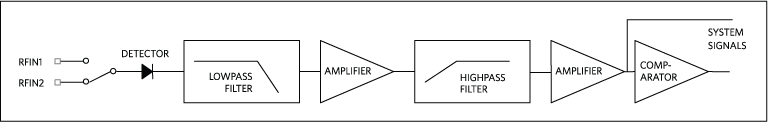RFIN Demodulation Inputs

RFIN1 and RFIN2 inputs have the same internal structure with the same sensitivity. They are connected to a switch where the state is defined by the option bit 14:

 Bit 14 Description 0 RFIN1 input selected 1 RFIN2 input selected

If one input is not used, it has to be connected to the analog ground through a 10nF capacitor.

Demodulator

The asynchronous detector removes the 13.56MHz carrier and transmits the amplitude modulation to the rest of the reception chain.

Filters

A lowpass and highpass filters form the reception chain filtering. It is possible through the chip option bits (bit 8, 9, and 10) to set their corner frequency. This setting depends on the subcarrier used by the tag.

For example:

• Subcarrier: 424kHz
• Lowpass filter selection: 1MHz
• Highpass filter zero: 200kHz

Amplifier - AGC

By option bit 15, the user has the possibility to enable or disable the AGC function. The AGC on/off selection depends mainly on the final application. The amplifier gain could be decreased up to 40dB. In case of a noisy environment, it could be useful to reduce the gain value keeping the same reading performances.

To get the maximum of performances, it is recommended to use the AGC amplifier as follows:

 Features Option Bits Description AGC attack mode selection 16 Attack always AGC decay mode selection 17 Fast decay AGC attack rate 18 to 19 ~19dB/µs AGC decay wait 20 to 21 ~44µs

Comparator

The final stage comparator provides the tag modulation on a system output. This digital signal is used by the internal processing machine to read the data sent by the tag.

### Summary

This application note provides the equations and basic design philosophy for antenna design with the MAX66300.